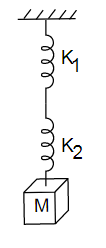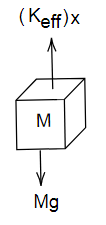Courses
Courses for Kids
Free study material
Free LIVE classes
More# A mass M is suspended by two springs of force constants ${K_1}$ and ${K_2}$ respectively as shown in the diagram. The total elongation (stretch) of the two springs isA. $\dfrac{{Mg}}{{{K_1} + {K_2}}} \\$B. $\dfrac{{Mg\left( {{K_1} + {K_2}} \right)}}{{{K_1}{K_2}}} \\$C. $\dfrac{{Mg\left( {{K_1}{K_2}} \right)}}{{{K_1} + {K_2}}} \\$D. $\dfrac{{{K_1} + {K_2}}}{{{K_1}{K_2}Mg}}$Verified
54.3k+ views
Hint: Force on the system will be a product of effective constant for the series combination and elongation in the spring.

Formula used:
The expression of restoring force is,
$F = Kx$
Where, F = Force, k= Spring constant and x = Elongation (stretch) of the spring.

Complete step by step solution:
Given here is a spring mass system of two springs having constant ${K_1}$ and ${K_2}$ respectively. Springs are combined together in series combination and a mass M is suspended by the springs, we have to find the elongation in springs.

First we need to find the effective constant for the given combination of springs and it will be,
${K_{eff}} = \dfrac{{{K_1}{K_2}}}{{{K_1} + {K_2}}}\,.........(1)$
Let the elongation in spring be x then free body diagram of suspended mass M will be,Image: Free body diagram of mass M

From free body diagram we have,
${K_{eff}}x = Mg \\ \Rightarrow x = \dfrac{{Mg}}{{{K_{eff}}}}\,.......(2)$
Substituting value of ${K_{eff}}$ form equation (1) in equation (2) we get,
$x = \dfrac{{Mg\left( {{K_1} + {K_2}} \right)}}{{{K_1}{K_2}}}\,$
Hence, elongation in the spring will be $\dfrac{{Mg\left( {{K_1} + {K_2}} \right)}}{{{K_1}{K_2}}}\,$.

Therefore, option B is the correct answer.

Note: Even though in combination of springs we have multiple springs connected to each other either in series or parallel, they behave like a single spring and to solve numerical problems for combination of springs their effective constant is to be calculated first.
Last updated date: 25th May 2023
Total views: 54.3k
Views today: 1.13k